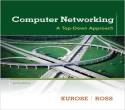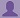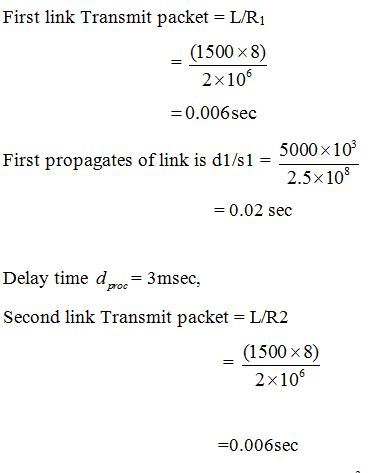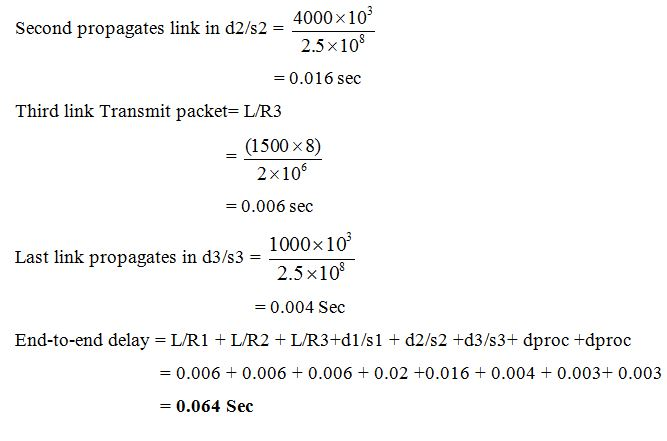SHARE
HELP

To start with, Sr2Jr’s first step is to reduce the expenses related to education. To achieve this goal Sr2Jr organized the textbook’s question and answers. Sr2Jr is community based and need your support to fill the question and answers. The question and answers posted will be available free of cost to all.# Computer Networking : A Top-down Approach

Authors:
James F. Kurose, Keith W. Ross
Exercise:
Problems
Chapter:
Computer Networks And The Internet
Edition:
6
ISBN:
9780132856201
Question:
10
 Previous Next

###Question

Consider a packet of length L which begins at end system A and travels over three links to a destination end system. These three links are connected by two packet switches. Let di, si, and Ri denote the length, propagation speed, and the transmission rate of link i, for i = 1, 2, 3. The packet switch delays each packet by dproc. Assuming no queuing delays, in terms of di, si, Ri, (I = 1,2,3), and L, what is the total end-to-end delay for the packet? Suppose now the packet is 1,500 bytes, the propagation speed on all three links is 2.5 · 108 m/s, the transmission rates of all three links are 2 Mbps, the packet switch processing delay is 3 msec, the length of the first link is 5,000 km, the length of the second link is 4,000 km, and the length of the last link is 1,000 km. For these values, what is the end-to-end delay?

###AnswerTherefore, the end-to-end delay =0.064 seconds

### Discussions

Post the discussion to improve the above solution.